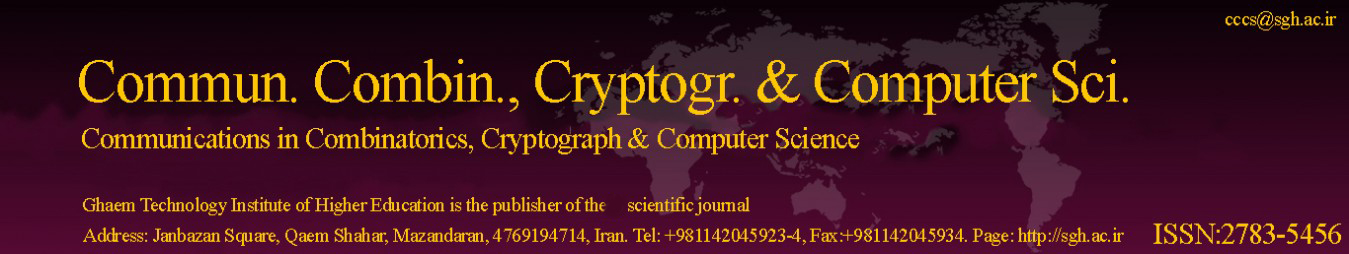Original Research Article

Article volume = 2021 and issue = 1

Pages: 27–32

Article publication Date: February 19, 2021

# The Total Irregular Labelling of Caterpillars with Q Internal Vertices of Degree Three for All Odd Number Q > 5

#### M. Riski Maulana, Isnaini Rosyida, Mulyono.

Department of Mathematics, Faculty of Mathematics and Natural Sciences, Universitas Negeri Semarang, Indonesia.

##### Abstract:

We restrict the graph G(V, E) has no loop and multiple edges, no direction on its edges, and connected. The labelling is mentioned as a “totally irregular total k-labelling” if the vertices and edges of G have different weights. The number k is said to be the total irregularity strength of G, symbolized as ts(G), if k is the minimum number such that G has “totally irregular total k-labelling”. We verify the ts of caterpillar graphs Sm,3,3, ,3,m having q internal vertices of degree 3 for odd number q > 5. The ts is: ts(Sm,3,3, ,3,m) = ceil( (2m+q-1) 2 ) for q > 5 and m > (q+5)2.

##### Keywords:

Totally irregular total k-labelling, ts, caterpillar graph, degree, internal vertex.

##### References:
•  A. Ahmad, M. Ibrahim, M.K. Siddiqui, On the total irregularity strength of generalized petersen graph, Math. Reports 18, bf 68(2), (2016), 197—204. 1
•  M. Baˇca, S. Jendrol’, K. Kathiresan, K. Muthugurupackiam, A. Semaniˇcov´a-Fenovcikova A, A survey of irregularity strength, Electron. Notes Discrete Math., 48, (2015), 19–26. 1, 1, 1
•  S. A. U. H. Bokhary, M. Imran, U. Ali, On the total irregularity strength of convex polytope graphs, Proyecciones (Antofagasta, On line), (2021), Preproof, 10 pages. 1
•  J.A. Gallian, A Dynamic survey of graph labeling, Electron. J. Combin #DS6, (2019), 553 pages. 1
•  D. Indriati, Widodo, I.E. Wijayanti, K.A. Sugeng, On Total Irregularity Strength of Double-Star and Related Graphs, Procedia Computer Science, 74, (2015), 118–123. 1
•  D. Indriati, Widodo, I. Rosyida, K.A. Sugeng, The construction of labelling and total irregularity strength of specified caterpillar graph, J. Phys Conf Ser., Ser.855, 012018, (2017), 7 pages. 1
•  D. Indriati, I. Rosyida, Widodo, On the total irregularity strength of caterpillar with each internal vertex has degree three, J. Phys Conf Ser., Ser. 1008,012042, (2018), 8 pages. 1
•  D. Indriati, Widodo, I.E. Wijayanti, K.A. Sugeng, I. Rosyida, Totally irregular total labeling of some caterpillar graphs, Electron. J. Graph Theory Appl. 8(2), (2020), 247-254. 1
•  J. Ivanˇco and S. Jendroˇl, Total edge irregularity strength of trees, Discuss. Math. Graph Theory. 26, (2006), 449–456. 1
•  A. M. Marr and W.D. Wallis, Magic graphs, Birkhauser-Springer, New York, (2013). 1
•  M. R. Maulana1, I. Rosyida, Mulyono, The total irregularity strength of caterpillars with odd number of internal vertices of degree three, J. Phys.: Conf. Ser., 1918, 042034, (2021), 7 pages. 1
•  C.C. Marzuki, A.N.M. Salman, and M. Miller, On the total irregularity strength of cycles and paths, Far East J. Math. Sci. 82(1), (2013), 1–21. 1
•  C. C. Marzuki and Roslaini, Total irregularity strength of m-copies of rhombus graph, J. Phys.: Conf. Ser., 1116, 022023, (2018), 1-6. 1
•  M. Naeem and M.K. Siddiqui, Total irregularity strength of disjoint union of isomorphic copies of generalized Petersen graph, Discrete Mathematics, Algorithms and Applications, 9(6), 1750071, (2017), 9 pages. 1
•  Nurdin, E.T. Baskoro, A.N.M. Salman, and N.N. Gaos, On the total vertex irregularity strength of trees, Discrete Math. 310, (2010), 3043–3048. 1
•  R. Ramdani, A.N.M. Salman, H. Assiyatun, A. Semanicov´a-Fenovcikova, and M. Baˇca, On the total irregularity strength of three families of graphs, Math. Comput. Sci. 9(2), (2015), 229–237. 1
•  I. Rosyida, Widodo, D Indriati, On total irregularity strength of caterpillar graphs with two leaves on each internal vertex, J. Phys. Conf. Ser., 1008, 12046, 9 pages, (2018). 1
•  I. Rosyida, Mulyono, D. Indriati. On totally irregular total labeling of caterpillars having even number of internal vertices with degree three, 2326, 020024, (2021), 9 pages. 1
•  M.K. Siddiqui, M. Imran, M. Ibrahim, Total irregularity strength for product of two paths, AKCE International Journal of Graphs and Combinatorics, In press, 2018, 14 pages. 1
•  E.W. Weisstein, Caterpillar, MathWorld–A Wolfram Web Resource, https://mathworld.wolfram.com/CaterpillarGraph.html, (2020). 1